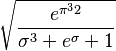Difference between revisions of "User:Vipul/Sandbox"$\sqrt{\frac{e^{\pi^32}}{\sigma^3 + e^\sigma + 1}}$